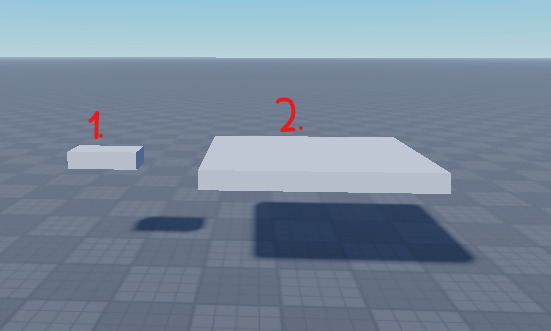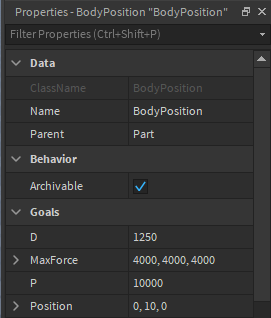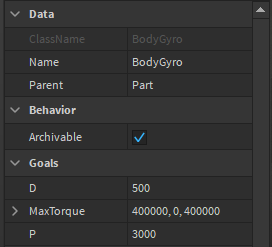# Bodymover - Massness problem

Hello, I’m new to Roblox physics. So I don’t know everything about Bodymovers.
I know how to move a part with Bodyposition + Bodygyro. I did. But there is a problem.

I made two part with the same Bodymovers, same properties. Here the parts:You see.

First part can move perfectly. But the second one can’t. These big parts creep like a snake or don’t move. I know you are gonna say “Massless” but Massless is true.
Can someone help me with that big parts?

Bodyposition:Bodygyro:Thanks for the reading2 Likes

You can get the mass of a Part by calling Part:GetMass(). Using the formula a = F/m, you can see that acceleration and mass are inversely proportional, so if mass doubles the acceleration halves. You don’t want it to halve, you want it to stay the same. So you need to double it again after halving. Force is proportional to acceleration, so you can double the acceleration again by doubling the force.

In other words, you need to multiply apply some amount of force per mass. You can achieve this by multiplying the force by the mass. MaxForce should probably be multiplied, as should `P` because that’s how much force to apply in proportion to how far the BodyPosition is from the target position.

The bodygyro is a bit harder, because AFAIK there’s no builtin- way to get the moment of inertia (the rotation equivalent of force) of parts in Roblox. Don’t really know how to go about thatBut you could try multiplying the MaxTorque and P of the BodyGyro by the mass as well

3 Likes

oh my god, I didn’t know bodymovers are that complex.

But I set it as Massless. Also do you know a source about this so I can learn it.